SLUS769D July   2013  – December 2016

PRODUCTION DATA.

1. Features
2. Applications
3. Description
4. Simplified Schematic
5. Revision History
6. Device Comparison Table
7. Pin Configuration and Functions
8. Detailed Pin Description
9. Specifications
10. 10Detailed Description
1. 10.1 Overview
2. 10.2 Functional Block Diagram
3. 10.3 Feature Description
4. 10.4 Device Functional Modes
11. 11Applications and Implementation
1. 11.1 Application Information
2. 11.2 Typical Application
1. 11.2.1 Battery Charger, 5 V, 6 W
1. 11.2.1.1 Design Requirements
2. 11.2.1.2 Detailed Design Procedure
2. 11.2.2 Application Curves
3. 11.2.3 Multi-Output Converter with UCC2891x Devices
4. 11.2.4 Do’s and Don'ts
12. 12Power Supply Recommendations
13. 13Layout
14. 14Device and Documentation Support
1. 14.1 Documentation Support
1. 14.1.1 Device Nomenclature
2. 14.1.2 Related Documents
3. 14.3 Electrostatic Discharge Caution
4. 14.4 Glossary
15. 15Mechanical, Packaging, and Orderable Information

• D|7

## 11 Applications and Implementation

### 11.1 Application Information

The UCC28910 and UCC28911 devices are HV switcher that integrates an HV power FET plus a controller that uses primary-side-regulated (PSR), supporting magnetically-sensed output voltage regulation via the transformer bias winding. This sensing eliminates the need for a secondary-side reference, error amplifier and optical-isolator for output voltage regulation. The devices deliver accurate output voltage static load and line regulation, and accurate control of the output current. The magnetic sampling scheme allows operation only in discontinuous conduction mode (DCM) so the device is not allowed to turn on the Power FET if it doesn’t sense a ZCD event. A ZCD event is when auxiliary winding voltage crosses zero from high to low after transformer demagnetization is completed. The modulator adjusts both frequency and peak current in different load regions to maximize efficiency throughout the operating range. The smart management of the control logic power consumption and the HV current source, used for startup that is off during operation and have very low leakage current, allow designing converters with very low standby input power. The less than 30mW can be easily achieved with these devices.

### 11.2 Typical Application

#### 11.2.1 Battery Charger, 5 V, 6 W

This design example describes the UCC28910FBEVM-526 design and outlines the design steps required to design a constant-voltage, constant-current flyback converter for a 5-V/6-W charger. Discontinuous conduction mode (DCM) with valley switching is used to reduce switching losses. A combination of switching frequency and peak primary current amplitude modulation is used to keep conversion efficiency high across the full load and input voltage range. Figure 33 below details the output V-I characteristic. Low system parts count and built in advanced protection features result in a cost-effective solution that meets stringent world-wide energy efficiency requirements.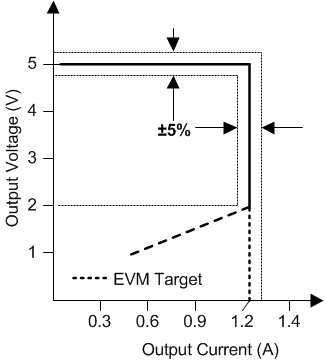Figure 33. Target Output V-I Characteristic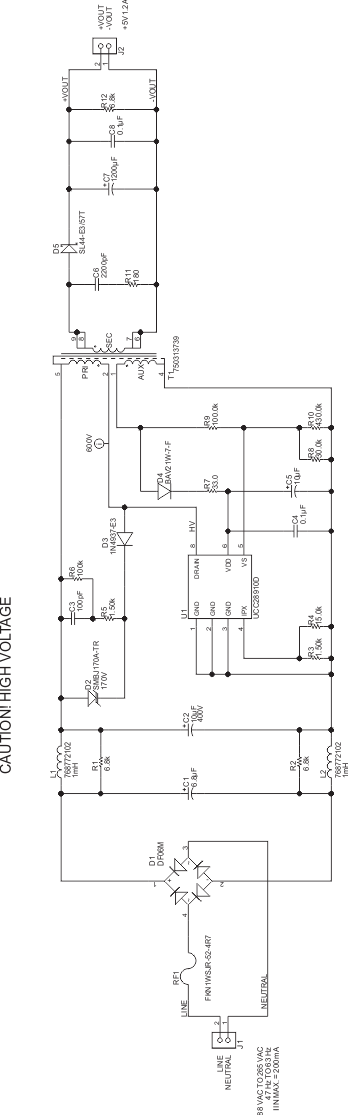Figure 34. UCC28910FBEVM-526 Schematic

#### 11.2.1.1 Design Requirements

For this design example, use the parameters listed in Table 1.

### Table 1. Design Parameters

PARAMETER TEST CONDITIONS MIN TYP MAX UNIT
INPUT CHARACTERISTICS
VIN Input voltage 90 115/230 265 V
fLINE Frequency 47 50/60 64 Hz
PNL No load power VIN = VNOM IOUT = 0 A 15 20 mW
VINUVLO Brownout voltage IOUT = INOM 70 V
VINOV Brownout recovery voltage 80 V
IIN Input current VIN = VMIN, IOUT = max 0.2 A
OUTPUT CHARACTERISTICS
VOUT Output voltage VIN =VMIN to VMAX, IOUT = 0 V to INOM 4.75 5.00 5.25 V
IOUT(max) Maximum output current VIN = VMIN to VMAX 1.14 1.20 1.26 A
IOUT(min) Minimum output current Vin = Vmin to VMAX 0 A
ΔVOUT Output voltage ripple VIN = VMIN to VMAX, IOUT = 0 V to INOM 150 mV
POUT Output power VIN = VMIN to VMAX
SYSTEM CHARACTERISTICS
η Average efficiency 25%, 50%, 75%, 100% of IOUT 75%
ENVIRONMENTAL
Conducted EMI Meets CISPR22B/EN55022B

#### 11.2.1.2 Detailed Design Procedure

This procedure outlines the steps to design a constant-voltage, constant-current flyback converter based on UCC28910 switcher. Refer to the Figure 35 for component names and network locations. The design procedure equations use terms that are defined below.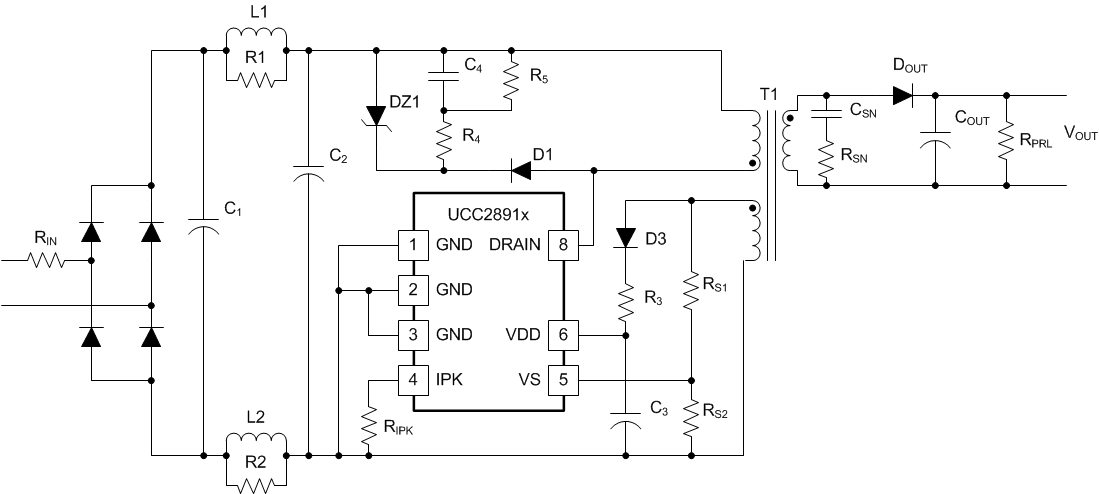Figure 35. Standard Flyback Converter Based on UCC2891x

#### 11.2.1.2.1 Power Handling Curves

The power handling curves give the maximum output power that can be handled by the devices versus the ambient temperature. These curves give the maximum output power achievable considering that the losses inside the device can cause rise of the junction temperature up to 125°C. The thermal resistance junction to ambient were provided with considerations to two different values. Thermal resistances values of 50°C /W and of 100°C / W were considered.

The device handling capability depends on the overall design and input and output voltage. Figure 36 and Figure 38 refer to a wide-range input voltage (90 VAC; 264 VAC) converter; Figure 37 and Figure 39 refer to a European range input voltage (180 VAC; 265 VAC). The dotted line curves refer to 12-V output voltage AC-to-DC converter, the continuous line curves refer to 5-V output voltage converter.

Figure 36 and Figure 37 show the power handling capabilities of UCC28910 and Figure 38 and Figure 39 show the power handling capabilities of UCC28911.

### Table 2. Maximum Output Power(3)

PART NUMBER 180 VAC to 265 VAC 90 VAC to 265 VAC UNITS
UCC28910 6.5 9.5 6 7.5 W
UCC28911 8 12 7.5 10 W
Typical continuous power in enclosed adapter at 50°C ambient, with adequate ( > 560 mm2) copper area connected on GND pin to have 90°C/W as junction to ambient thermal resistance.
Maximum continuous power with open frame design at 50°C ambient, with adequate copper area connected on GND pin and/or adequate air flow to have 50°C/W as junction to ambient thermal resistance.
Table 2 is obtained considering 125°C as maximum junction temperature. For lower operating maximum junction temperature, the maximum output power should be lower.

The curves provided show the maximum power obtained through optimized designs.

A lower-efficiency design requires the converter to process more input power to deliver the same amount of power to the load. Therefore, less power handling capability is expected for lower-efficiency designs.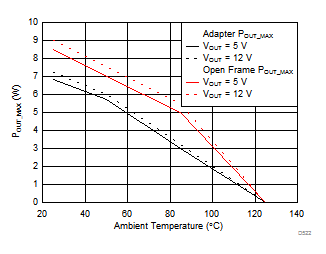Figure 36. [90; 264] VRMS Input Voltage Range,
(maximum input power vs ambient temperature of UCC28910)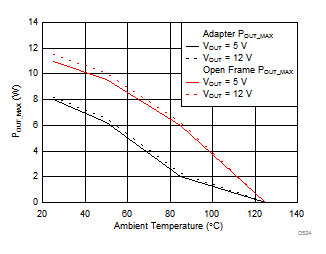Figure 38. 90 VRMS, 264 VRMS Input Voltage Range,
(maximum output power vs ambient temperature of UCC28911)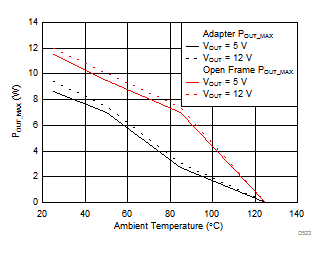Figure 37. [180; 264] VRMS Input Voltage Range,
(maximum input power vs ambient temperature of UCC28910)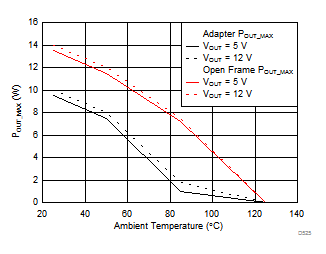Figure 39. 180 VRMS, 264 VRMS Input Voltage Range,
(maximum output power vs ambient temperature of UCC28911)

#### 11.2.1.2.2 Input Stage Design and Bulk Capacitance

The input stage consists of:

1. Input fuse resistor (RIN) is generally used to:
1. Limit the inrush current on the input capacitor when the line voltage is applied.
2. Disconnect the line in case of input over current.
2. A bridge diode or a single-wave rectifier diode used to rectify the main voltage.
3. A bulk capacitor (capacitors C1 and C2) that stores the energy and reduces input voltage ripple.
4. A line filter (L1, L2, R1, and R2) to reduce EMI generated by switching.

The minimum input capacitance voltage, the input power of the converter based on target full-load efficiency, minimum input RMS voltage, and minimum AC input frequency are used to determine the input capacitance requirement.

The maximum converter input power can be estimated from the output voltage in voltage constant mode, VOCV, the converter's output current when operating in constant current more, IOCC, and the full-load efficiency target, η.

Equation 6.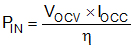The following equation provides an accurate solution for input capacitance based on a target minimum bulk capacitor voltage. To target a given input capacitance value, iterate the minimum capacitor voltage to achieve the target capacitance.

Equation 7.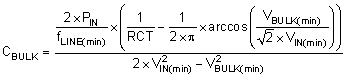In the case where the input rectifier is a single diode (half wave rectifier) , and for applications with bridge input rectifier (full wave rectifier), as in the schematic of Figure 31.

The voltage VBULK(min) is generally selected around 65% to 60% of √2 x VIN(min). VBULK(min) is determined by the selection of the high-voltage input capacitors.

For the considered example we have:

Equation 8.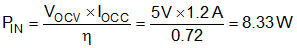Equation 9.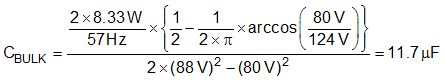Taking into account that electrolytic capacitance, with 20% of tolerance, the values selected for C1 and C2 are: C1 = 6.8 μF and C2 = 10 μF.

#### 11.2.1.2.3 Transformer Turns Ratio

The maximum primary-to-secondary turns ratio can be determined by the target maximum switching frequency at full load, the minimum input capacitor bulk voltage, and the estimated DCM quasi-resonant time. Initially determine the maximum available total duty cycle of the on-time and secondary conduction time based on target switching frequency and DCM resonant time. For DCM resonant time, assume tR = 1 / 500 kHz if you do not have an estimate from previous designs. For the transition mode operation limit, the period required from the end of secondary current conduction to the first valley of the VDS voltage is half of the DCM resonant period, or 1 μs assuming 500-kHz resonant frequency. DMAX can be determined using the equation below.

Equation 10.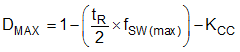Where KCC is the regulation gain in constant current control mode and is equal to the secondary diode conduction duty cycle when the converter is operating at maximum output current.

Once DMAX is known, the maximum turn ratio of the primary-to-secondary can be determined with the equation below.

Equation 11.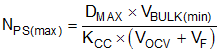The total voltage on the secondary winding needs to be determined; the sum of VOCV and the secondary rectifier VF. The voltage VBULK(min) is generally selected around 65% or 60% of the peak of low-line. VBULK(min) is determined by the selection of the high-voltage input capacitors.

For the 5-V USB charger applications NPS values from 13 to 17 are typically used.

For our example the maximum value for primary to secondary turn ratio will be:

Equation 12.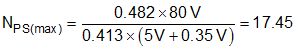In this example we fix NPS = 16.5

In order to calculate the primary to auxiliary turn ratio (NPA) we use the parameter VOCC(min) that is the minimum output voltage at which we want to guarantee the output current regulation.

Equation 13.In the example:

Equation 14.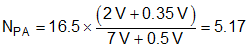In Equation 13 VFAUX is the D3 diode voltage drop when conducting. The value of NPA is generally under-estimated and the number of auxiliary winding turns can be reduced with respect to the value provided by Equation 13. Optimization can be done directly on the circuit verifying the margin between the VDD's measured value and VDDOFF(max).

#### 11.2.1.2.4 Output Capacitance

The output capacitance value is typically determined by the transient response requirement from no load. For example, in USB charger applications, it is often required to maintain a minimum output voltage of 4.1 V with a load-step transient from 0 mA to 500 mA (ITRAN). The equation below assumes that the switching frequency is at the minimum of fSW(min).

Equation 15.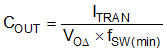Equation 16.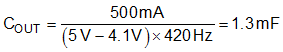Another consideration on the output capacitor(s) is the ripple voltage requirement which is reviewed based on secondary-peak current and ESR. A margin of 20% is added to the capacitor ESR requirement in the equation below.

Equation 17.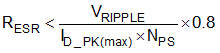VRIPPLE is the maximum output-voltage ripple allowed for the design. The UCC2891x devices incorporates internal voltage-loop compensation circuits so that external compensation is not necessary, provided that the value of COUT is high enough. The following equation determines a minimum value of COUT necessary to maintain a phase margin >30 degrees over the full-load range.

Equation 18.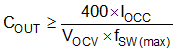#### 11.2.1.2.5 VDD Capacitance, CVDD

The capacitance on VDD needs to supply the device operating current until the output of the converter reaches the target minimum operating voltage in constant-current regulation. At this time the auxiliary winding can sustain the supply voltage. The output current available to the load to charge the output capacitors is the constant-current regulation target. The equation below assumes the output current of the flyback is available to charge the output capacitance until the minimum output voltage is achieved. CVDD capacitor is the C3 capacitor in the schematic of Figure 35.

Equation 19.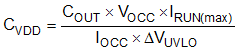#### 11.2.1.2.6 VS Resistor Divider

The VS divider resistors determine the output voltage regulation point of the flyback converter, also the high-side divider resistor (RS1) determines the line voltage at which the controller enables continuous switching operation. RS1 is initially determined based on transformer auxiliary to primary turn ratio and desired input voltage operating threshold.

Equation 20.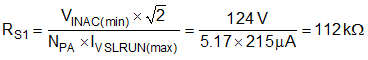In our example the selected value of RS1 was 100 kΩ.

The low-side VS pin resistor is selected based on desired output voltage regulation.

Equation 21.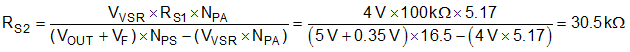The value selected for RS2 resistance was 30 kΩ.

#### 11.2.1.2.7 RVDD Resistor and Turn Ratio

The value of RVDD and the auxiliary-to-secondary turns ratio should be selected with care in order to be sure that the VDD is always higher than the VDDOFF (7 V maximum) threshold under all operating conditions. The RVDD resistor also limits the current that can go into the VDD pin preventing IVDDCLP_OC clamp over-current protection from being erroneously activated.

#### 11.2.1.2.8 Transformer Input Power

The power at the transformer input during full-load condition is given by the output power plus the power loss in the output diode plus the power consumption of the UCC2891x control logic (VVDD × IRUN) divided by the transformer efficiency that takes into account all the losses due to the transformer: copper losses, core losses, and energy loss in the leakage inductances.

Equation 22.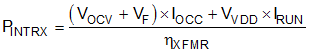#### 11.2.1.2.9 RIPK Value

The RIPK value sets the value of the DRAIN current peak that equals the transformer primary winding current peak value. This value also sets the value of the output current when working in CC mode according to the following formula:

Equation 23.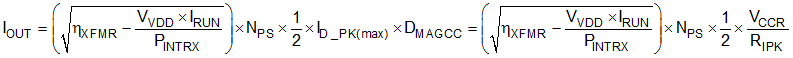where

The term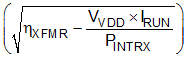takes into account that not all the energy stored in the transformer goes to the secondary side but some of this energy, through the auxiliary winding, is used to supply the device control logic. The transfer of energy always happens with unavoidable losses. These losses are accounted for through the transformer efficiency term (ηXFMR). For a fixed target value for IOUT, the value of RIPK can be calculated using the following formula:

Equation 24.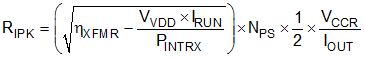For the example:

Equation 25.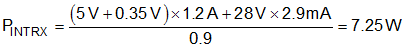Equation 26.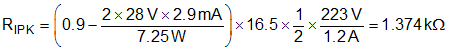#### 11.2.1.2.10 Transformer Primary Inductance Value

After you have fixed the maximum switching frequency and the maximum value of the primary current peak for your application, the primary inductance value can be fixed by the following equation:

Equation 27.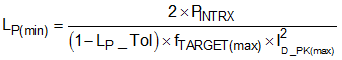LP_Tol is the tolerance on the primary inductance value of the transformer. Typical values of LP_Tol are between ±10% and ±15%)

ID_PK(max) is given by:

Equation 28.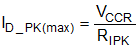For the example:

Equation 29.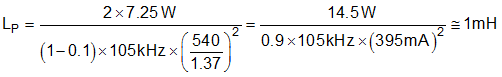#### 11.2.1.2.10.1 Secondary Diode Selection

The maximum reverse voltage that the secondary diode had to sustain can be calculated by the equation below where a margin of 30% is considered. Usually for this kind of application a Schottky diode is used to reduce the power losses due to the lower forward voltage drop. The maximum current rating of the diode is generally selected between two and five times the maximum output current (IOCC).

Equation 30.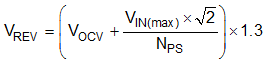Equation 31.When no load is applied on the converter output, the output voltage rises until the OVP (over voltage protection) of the device is tripped, because the device cannot operate at zero switching frequency. The device's minimum switching frequency of 420 Hz will always deliver some energy to the output, causing the voltage to rise at no load. To avoid this, an RPRL (pre-load resistance) is used. The value of this pre-load can be selected using the following equation:

Equation 32.Equation 33.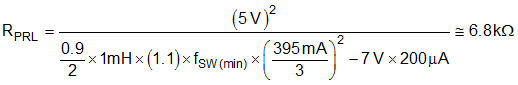#### 11.2.1.2.12 DRAIN Voltage Clamp Circuit

The main purpose of this circuit, as in most flyback converters, is to prevent the DRAIN voltage from rising up to the FET break-down voltage, at the FET turn-off, and destroying the FET itself. An additional task, required by the primary-side regulation mechanism, is to provide a clean input to the VS pin by damping the oscillation that is typically present on the DRAIN voltage due to the transformer primary leakage inductance.

To perform damping, the D2 diode (refer to Figure 40) selected should not be a fast recovery diode (0.3 μs < trr < 1 μs) so the reverse current can flow in the RLC over damped circuit. This RLC circuit is formed by the transformer primary-leakage inductance (LLKP), the resistance R4, and the capacitance C4. To ensure proper damping the resistance R4 has to satisfy the following condition:

Equation 34.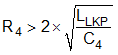The capacitance C4 should not be too high so it does not require too much energy to be charged. Typical values for C4 are between 100 pF and 1 nF.

The resistance, R5, has been added to discharge the C4 capacitance so at the next switching cycle diode D2 is activated providing enough current and storage to have a reverse-recovery current large enough for proper oscillation damping.Figure 40. DRAIN Clamp Circuit Options

#### 11.2.2 Application Curves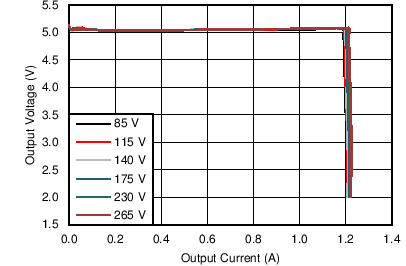Figure 41. Output V-I CharacteristicFigure 43. Output V-I Characteristic Output Voltage Regulation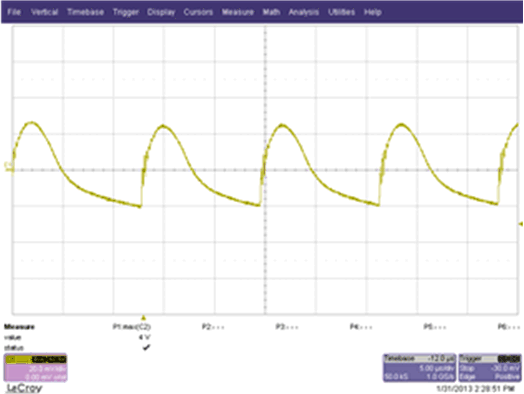Figure 45. Ripple with 5-V, 1.2-A Output, 85 VAC Input,
20 mV/div, 5 µs/div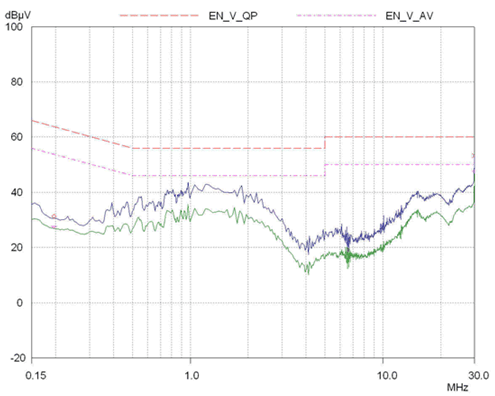Figure 47. EMI Test Results per EN55022, Class B. 115 VAC Input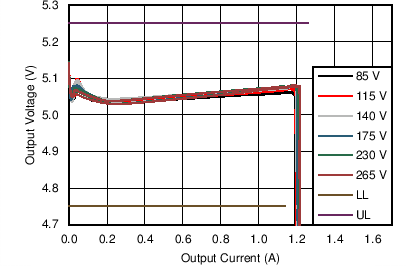Figure 42. Output V-I Characteristic Output Current RegulationFigure 44. Efficiency vs POUT Diagram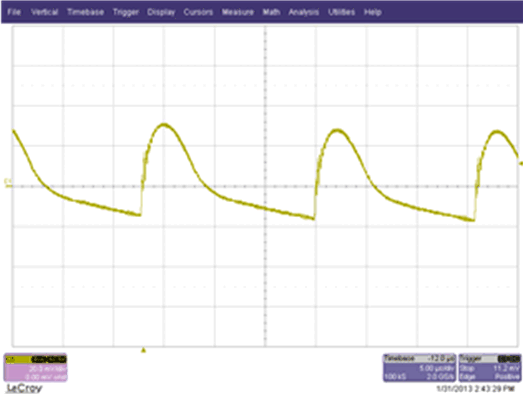Figure 46. Ripple with 5-V,1.2-A Output, 265-VAC Input,
20 mV/div 5 µs/div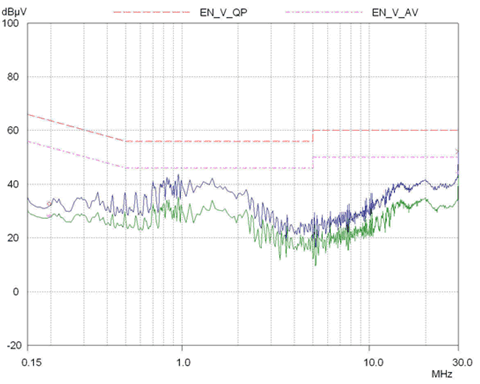Figure 48. EMI Test Results per EN55022, Class B. 230-VAC Input

#### 11.2.2.1 Average Efficiency Performance and Standby Power of the UCC28910FBEVM-526

Table 3 summarizes the average efficiency performance of the UCC28910FBEVM-526 and Table 4 summarizes the standby power that is the no-load power consumption of the converter.

### Table 3. Average Efficiency Performance of the UCC28910FBEVM-526

VIN (VAC) f (Hz) PIN (W) IOUT (A) VOUT (V) POUT (W) EFFICIENCY (%) AVERAGE EFFICIENCY (%)
115 60 7.826 1.201 4.950 5.943 75.94 76.25
5.845 0.901 4.942 4.451 76.15
3.889 0.601 4.934 2.964 76.19
1.930 0.301 4.927 1.481 76.73
230 50 7.721 1.201 4.956 5.950 77.06 76.68
5.783 0.901 4.948 4.457 77.07
3.853 0.601 4.938 2.966 76.97
1.960 0.301 4.930 1.482 75.60

### Table 4. Standby Power, No-Load Power Consumption of the Converter

VIN (VAC) f (Hz) PIN (mW)
88 60 10
115 60 10
230 50 10
265 50 12

#### 11.2.3 Multi-Output Converter with UCC2891x Devices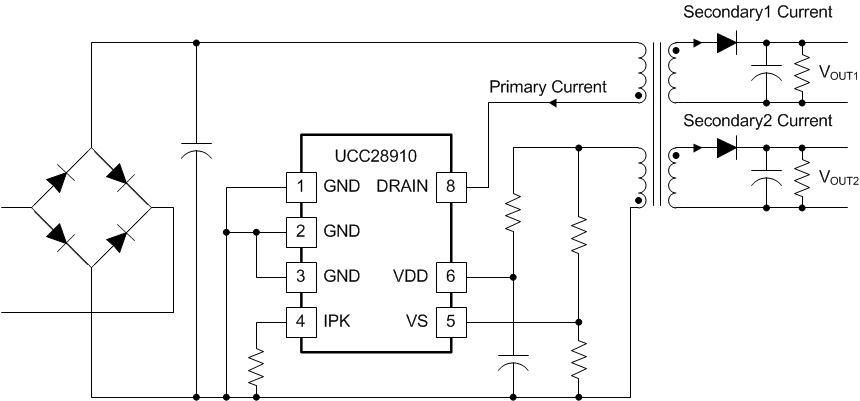Figure 49. Multi-Output Flyback Converter

UCC2891x devices perform the output voltage regulation through PSR (Primary-Side Regulation). With PSR the output voltage is sensed sampling the auxiliary winding at the end of the transformer demagnetization. With multi-output flyback that implies multiple-secondary windings, the demagnetization time is not uniquely defined. We have multiple demagnetization time, one for each secondary winding. If secondary windings are well coupled together the UCC2891x samples the auxiliary winding at the end of the last demagnetization. (See Figure 50).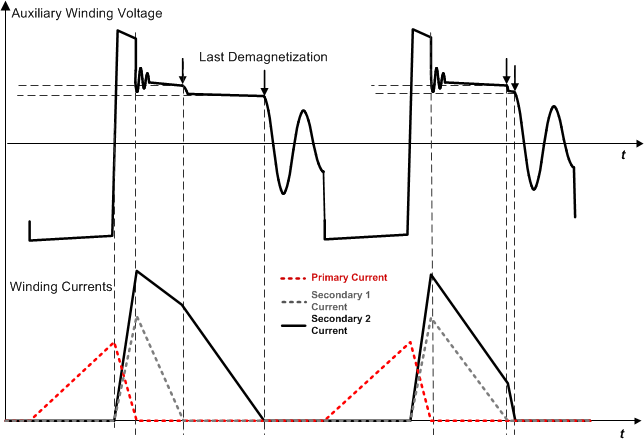Figure 50. Winding Current Waveform in Multi-Output Flyback Converter

In multiple output flyback converter regardless if they use primary-side regulation or secondary-side regulation, the output voltages are not regulated at the target values in all the load ranges. This is called a cross-regulation problem. To minimize this problem, if the two outputs refer to the same ground voltage, the output stage can be configured as a DC stacked output, instead of staked windings (see Figure 51).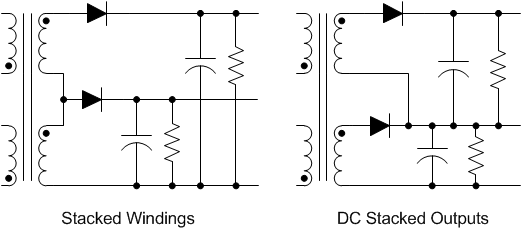Figure 51. Stacked Windings and DC Stacked Outputs

#### 11.2.4 Do’s and Don'ts

Always design the converter to operate at maximum switching frequency (see fSW(max) in Electrical Characteristics).

Select the RIPK to meet maximum output current requirement according to equation provided as first steps and fine tune the value on the real circuit according to design specification.

Provide enough copper area, connected to GND pins, to provide heat sinking capabilities.

Design the converter to keep junction temperature below 125°C in the worst case condition (maximum ambient temperature, minimum input voltage and maximum load). Estimate the junction temperature by measuring the GND pins temperature. The GND pins temperature are between 5°C to 15°C lower than the junction temperature.